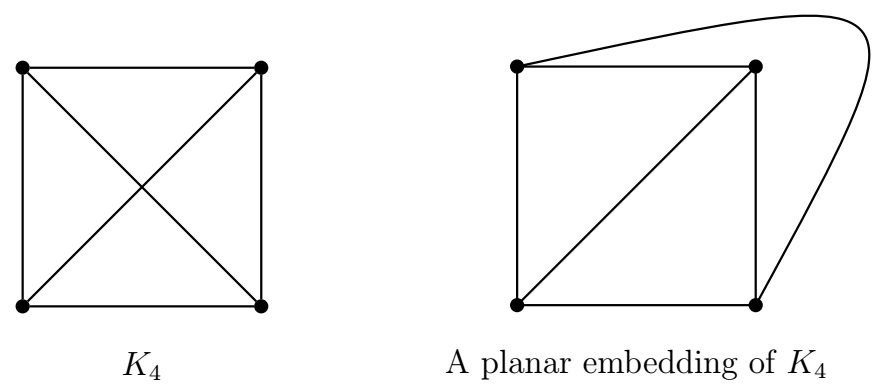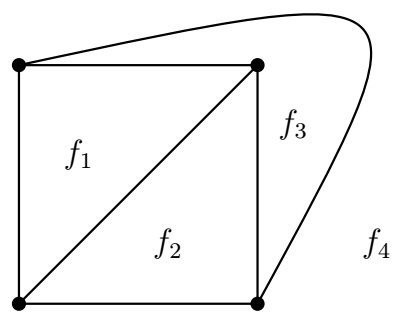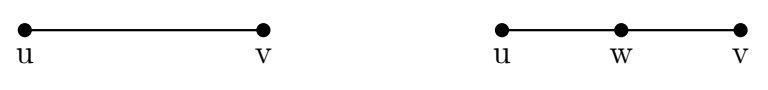Discrete Math Home

## Planar Graphs

Can we join three houses with three utilities so that none of the connections crosses? Note that the corresponding graph is $$K_{3,3}$$. So can we draw $$K_{3,3}$$ without edge-crossing?

Definition (Planar graph). A graph is planar if it can be drawn in the plane such that its edges intersect only at their endpoints (i.e., edges do not cross each other). A graph with such a drawing is called a planar embedding of the planar graph.

Example. $$K_4$$ is a planar graph. $$K_5$$ and $$K_{3,3}$$ are not planar.Definition (Face). A planar embedding of a planar graph splits the plane into regions called faces including an unbounded region called the outer face.

Example. The following planar embedding of $$K_4$$ has 4 faces where face $$f_4$$ is the outer face.Theorem (Euler's formula). Let $$G$$ be a simple connected planar graph on $$v$$ vertices and $$e$$ edges. If $$f$$ is the number of faces in a planar embedding of $$G$$, then $v-e+f=2.$

By induction on $$e$$, the number of edges of $$G$$.

Example. For $$K_4$$, we have $$v=4$$ and $$e=\binom{4}{2}=6$$. By Euler's formula, the number of faces in a planar embedding of $$K_4$$ is $f=e-v+2=6-4+2=4.$
Problem. Find the number of faces in a planar embedding of a $$3$$-regular connected planar graph $$G$$ on 8 vertices.

Solution. Here $$v=8$$ and the degree-sum is $$3\cdot 8=24$$. So $e=\frac{1}{2}(\text{the degree-sum})=\frac{1}{2}\cdot 24=12.$ By Euler's formula, the number of faces in a planar embedding of $$G$$ is $f=e-v+2=12-8+2=6.$
Theorem. Let $$G$$ be a simple connected planar graph on $$v\geq 3$$ vertices and $$e$$ edges. Then the following hold.

1. $$e\leq 3v-6$$, and

2. $$e\leq 2v-4$$ when $$G$$ contains no $$C_3$$.

Let $$f$$ be the number of faces in a planar embedding of $$G$$.

(a) The degree of a face $$f_i$$, denoted by $$\operatorname{d}(f_i)$$, is defined to be the number of edges on the boundary of $$f_i$$. Since $$v\geq 3$$, the degree of the outer face is at least 3. Since $$G$$ has no loops or multiedges, $$\operatorname{d}(f_i)\geq 3$$ for each face $$f_i$$. Note that each edge is counted twice in the degree-sum of faces. Thus $2e=\sum_{\text{face } f_i}\operatorname{d}(f_i).$ Since $$\operatorname{d}(f_i)\geq 3$$ for each face $$f_i$$, we have $2e=\sum_{\text{face } f_i}\operatorname{d}(f_i)\geq 3f.$ Thus $$f\leq \frac{2}{3}e$$. By Euler's formula, $$e-v+2=f\leq \frac{2}{3}e$$. Thus $$\frac{1}{3}e\leq v-2$$ which implies $$e\leq 3v-6$$.

(b) Similar proof.

Problem. Use an upper bound of the number of edges of a planar graph to prove that $$K_5$$ and $$K_{3,3}$$ are not planar graphs.

Solution. For $$K_5$$, $$v=5$$ and $$e=\binom{5}{2}=10$$. Suppose that $$K_5$$ is planar. Then by the above theorem, $$10=e\leq 3v-6=3\cdot5-6=9$$, a contradiction. Thus $$K_5$$ is not a planar graph.

For $$K_{3,3}$$, $$v=6$$ and $$e=3\cdot3=9$$. Since $$K_{3,3}$$ is a bipartite graph, it has no odd cycles. Suppose that $$K_{3,3}$$ is planar. Then by the above theorem, $$9=e\leq 2v-4=2\cdot6-4=8$$, a contradiction. Thus $$K_{3,3}$$ is not a planar graph.

Problem. Prove that if $$G$$ is a simple connected planar graph, then $$G$$ has a vertex of degree at most 5.

Solution. Let $$v$$ and $$e$$ be the number of vertices and edges of a simple connected planar graph $$G$$ respectively. If $$v=1 \text{ or } 2$$, then the result is true. Suppose $$v\geq 3$$. Then by (a) of the preceding theorem, $$e\leq 3v-6$$. Suppose $$\operatorname{d}(x)\geq 6$$ for all vertices $$x$$ of $$G$$. Then $e=\frac{1}{2}\sum_{\text{vertex }x}\operatorname{d}(x)\geq \frac{1}{2}(6v)=3v$ which contradicts the fact that $$e\leq 3v-6 < 3v$$.

Definition (Subdivision). A subdivision of an edge $$\{u,v\}$$ is the path $$uvw$$ obtained by inserting a new vertex $$w$$ on the edge $$\{u,v\}$$. A subdivision of a graph $$G$$ is obtained from $$G$$ by subdivision of edges in $$G$$.Remark. A graph $$G$$ is planar if and only if every subdivision of $$G$$ is planar.

Theorem (Kuratowski, 1930). A graph is planar if and only if it does not contain a subdivision of $$K_5$$ or $$K_{3,3}$$.

($$\Longrightarrow$$) Since $$K_5$$ or $$K_{3,3}$$ are nonplanar, their subdivisions are also nonplanar. Thus if a graph is planar, then it does not contain a subgraph that is a subdivision of $$K_5$$ or $$K_{3,3}$$.
($$\Longleftarrow$$) Nontrivial proof.

Remark. Applications of planar graphs include designing road networks without underpasses or overpasses and designing electronic circuits on a board without insulated wires. There are interesting topics related to planar graphs such as thickness, crossing number, graph embedding on surfaces (see topological graph theory).

Last edited